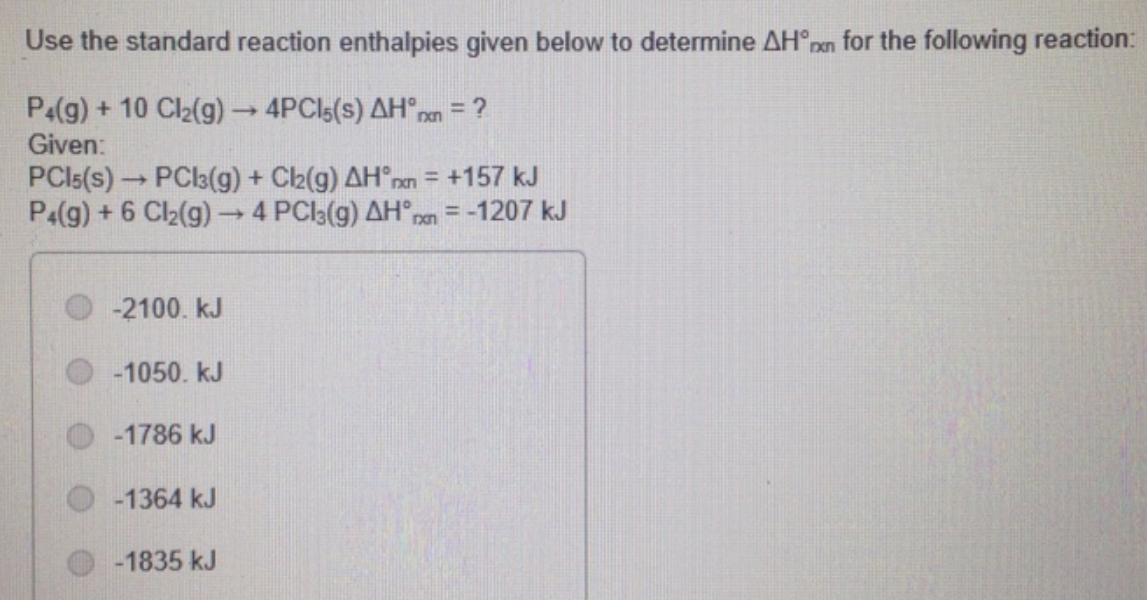# Problem: Use the standard reaction enthalpies given below to determine ∆Hrxn for the following reactionP4 (g) + 10 Cl2(g) ---&gt; 4PCI5(s)  ∆Hrxn = ?GivenPCI5(s) --&gt; PCl5(s) + Cl2(g) ∆Hrxn = +157 kJP4(g) + 6 Cl2(g) --&gt; 4 PCl3(g) ∆Hrxn = -1207 kJ-2100. kJ-1050. kJ-1786 kJ-1364 kJ-1835 kJ

###### FREE Expert Solution
91% (200 ratings)###### Problem Details

Use the standard reaction enthalpies given below to determine ∆Hrxn for the following reaction

P4 (g) + 10 Cl2(g) ---> 4PCI5(s)  ∆Hrxn = ?

Given

PCI5(s) --> PCl5(s) + Cl2(g) ∆Hrxn = +157 kJ
P4(g) + 6 Cl2(g) --> 4 PCl3(g) ∆Hrxn = -1207 kJ

-2100. kJ
-1050. kJ
-1786 kJ
-1364 kJ
-1835 kJFrequently Asked Questions

What scientific concept do you need to know in order to solve this problem?

Our tutors have indicated that to solve this problem you will need to apply the Hess's Law concept. You can view video lessons to learn Hess's Law. Or if you need more Hess's Law practice, you can also practice Hess's Law practice problems.

What professor is this problem relevant for?

Based on our data, we think this problem is relevant for Professor Vartia's class at KU.